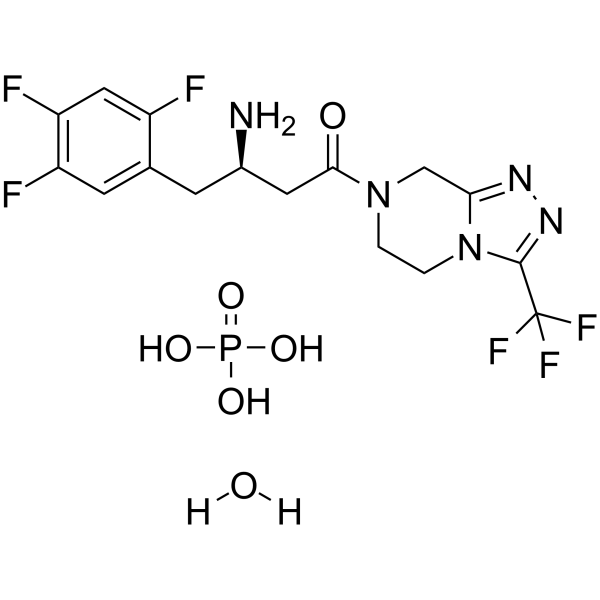# Sitagliptin phosphate monohydrate

Catalog No. T0242L   CAS 654671-77-9
Synonyms: MK-0431 phosphate monohydrate, 西他列汀磷酸盐一水合物, MK-0431

Sitagliptin phosphate is a potent inhibitor of DPP-IV with IC50 of 19 nM in Caco-2 cell extracts.

All products from TargetMol are for Research Use Only. Not for Human or Veterinary or Therapeutic Use.Sitagliptin phosphate monohydrate, CAS 654671-77-9
Product consultation
Get quote
Purity: 98%
Biological Description
Chemical Properties
Storage & Solubility Information
 Description Sitagliptin phosphate is a potent inhibitor of DPP-IV with IC50 of 19 nM in Caco-2 cell extracts. Targets&IC50 DPP-4:19 nM In vitro The IC50 of Nelarabine is 25-fold and 113-fold higher than ARAC in T- and B-lineage, respectively. T-ALL cells are eightfold more sensitive to Nelarabine than B-lineage but there is considerable overlap. The efficacy of NEL in T-lineage and B-lineage cell lines is 25-fold and 113-fold less than ARAC, respectively.  Nelarabine acts by inhibiting DNA synthesis and inducing apoptosis in susceptible cells. Nelarabine demonstrated significant antineoplastic activity with acceptable toxicity. 
 Synonyms MK-0431 phosphate monohydrate, 西他列汀磷酸盐一水合物, MK-0431 Molecular Weight 523.32 Formula C16H15F6N5O·H3PO4·H2O CAS No. 654671-77-9

#### Storage

Powder: -20°C for 3 years

In solvent: -80°C for 2 years

#### Solubility Information

H2O: 38 mg/mL (72.6 mM)

Ethanol: <1 mg/mL

DMSO: 93 mg/mL (177.7 mM)

( < 1 mg/ml refers to the product slightly soluble or insoluble )

## Related Products

Related compounds with same targets

##Dose Conversion

You can also refer to dose conversion for different animals. More

##In vivo Formulation Calculator (Clear solution)

Step One: Enter information below
Dosage
mg/kg
Average weight of animals
g
Dosing volume per animal
ul
Number of animals
Step Two: Enter the in vivo formulation
% DMSO
%
% Tween 80
% ddH2O

##Calculator

Molarity Calculator
Dilution Calculator
Reconstitution Calculation
Molecular Weight Calculator
=
X
X

### Molarity Calculator allows you to calculate the

• Mass of a compound required to prepare a solution of known volume and concentration
• Volume of solution required to dissolve a compound of known mass to a desired concentration
• Concentration of a solution resulting from a known mass of compound in a specific volume
See Example

An example of a molarity calculation using the molarity calculator
What is the mass of compound required to make a 10 mM stock solution in 10 ml of water given that the molecular weight of the compound is 197.13 g/mol?
Enter 197.13 into the Molecular Weight (MW) box
Enter 10 into the Concentration box and select the correct unit (millimolar)
Enter 10 into the Volume box and select the correct unit (milliliter)
Press calculate
The answer of 19.713 mg appears in the Mass box

X
=
X

### Calculator the dilution required to prepare a stock solution

Calculate the dilution required to prepare a stock solution
The dilution calculator is a useful tool which allows you to calculate how to dilute a stock solution of known concentration. Enter C1, C2 & V2 to calculate V1.

See Example

An example of a dilution calculation using the Tocris dilution calculator
What volume of a given 10 mM stock solution is required to make 20ml of a 50 μM solution?
Using the equation C1V1 = C2V2, where C1=10 mM, C2=50 μM, V2=20 ml and V1 is the unknown:
Enter 10 into the Concentration (start) box and select the correct unit (millimolar)
Enter 50 into the Concentration (final) box and select the correct unit (micromolar)
Enter 20 into the Volume (final) box and select the correct unit (milliliter)
Press calculate
The answer of 100 microliter (0.1 ml) appears in the Volume (start) box

=
/

### Calculate the volume of solvent required to reconstitute your vial.

The reconstitution calculator allows you to quickly calculate the volume of a reagent to reconstitute your vial.
Simply enter the mass of reagent and the target concentration and the calculator will determine the rest.

g/mol

### Enter the chemical formula of a compound to calculate its molar mass and elemental composition

Tip: Chemical formula is case sensitive: C10H16N2O2 c10h16n2o2

Instructions to calculate molar mass (molecular weight) of a chemical compound:
To calculate molar mass of a chemical compound, please enter its chemical formula and click 'Calculate'.
Definitions of molecular mass, molecular weight, molar mass and molar weight:
Molecular mass (molecular weight) is the mass of one molecule of a substance and is expressed n the unified atomic mass units (u). (1 u is equal to 1/12 the mass of one atom of carbon-12)
Molar mass (molar weight) is the mass of one mole of a substance and is expressed in g/mol.

bottom

## Tech Support

Please see Inhibitor Handling Instructions for more frequently ask questions. Topics include: how to prepare stock solutions, how to store products, and cautions on cell-based assays & animal experiments, etc.